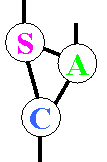# SA-C Overview## The SA-C Language: restricting C

Single Assignment C (SA-C) [pronounced "sassy"] is a variant of the C programming language that can be directly and intuitively mapped onto circuits, including FPGAs. A new language is necessary because C assumes a one-to-one correspondence between variables and memory locations. As a result, programmers can take the address of any variable and explicitly dereference pointers. In SA-C, on the other hand, variables correspond to wires in a circuit, and the address-of operator (&) and dereferencing operator (*) have been removed. More importantly, SA-C is a single assignment language, implying that the value of a variable can only be set once. Again, this is because variables in SA-C correspond to wires, and electrical wires can be driven by only one source. By comparison, in C a variable is a memory location whose value can be set and reset throughout the course of an algorithm.

The C language also assumes that programs are implemented on a von Neuman style calling stack. As a result, functions can recursively call each other. This is not possible in a circuit, which is a fixed-size piece of hardware. SA-C therefore bans recursion.

## The SA-C Language: Extending C

Having restricted C, we needed to add new features to compensate, and to exploit features of FPGAs not found in traditional processors. Most of the extensions were added with image and signal processing applications in mind, although many other applications may benefit from SA-C as well.

In image and signal processing, one of the most common use of pointers is to index into large arrays of data. SA-C obviates this use of pointers by adding true multidimensional arrays to the language, including dynamic arrays. For example, int32 arr[:,100] declares a two-dimensional array with one hundred columns; the number of rows is not specified. SA-C also introduces a parallel looping mechanisms that is tightly integrated with its multidimensional arrays. For example, for window w[3:3] in arr{...} will execute the loop body once for every possible 3x3 subwindow of the two-dimensional array arr.

SA-C also exploits the flexibility of FPGAs by introducing variable bit-precision data types. Whereas integers in C are limited to short, standard or long, SA-C programmers can specify the width of any variable in bits. In the example above, int32 declared a traditional 32-bit integer. However, we could also have written int12 or uint6 (the latter being an unsigned integer). SA-C also introduces fixed-point data types, allowing programmers to avoid building complex circuits for floating point arithmetic whenever possible.

Finally, SA-C compensates for the inconvenience of the single assignment restriction by allowing variable names to be reused, and by converting many C statements into expressions. New variables can be declared at any point in a SA-C program, and variables are initialized when they are declared (to enforce the single assignment restriction). To avoid a proliferation of variable names, however, SA-C programs can reuse previously used variable names. When a new variable is declared with the same name as a previous variable, the previous variable goes out of scope. As a result, statements such as int12 x = x + 1; are perfectly valid in SA-C. It simply declares a new variable x that replaces the old variable x (the new and old variables x may or may not have the same data type).

## Pragmas & Generics

In addition to the SA-C language itself, two other facilities enhance the SA-C programming environment. One is a pragma facility that allows users to control compiler optimizations. For example, users can turn on or off optimizations such as loop unrolling, stripmining, and implementing functions as lookup tables. This gives programmers control over how their programs are mapped onto circuits. The second facility is a Generic facility in the preprocessor. This facility allows code to be replicated for different data types, almost like a very simple version of C++'s template facility. It is added because variable bit resolution implies that SA-C has many more data types than traditional languages. As a result, it is often helpful to be able to implement a single library routine for many bit resolutions.

## A SA-C Example

A canonical image processing operation is to compute the magnitude of the edges in an image using horizontal and vertical edge masks. In our example, we use Prewitt edge masks. The basic algorithm is simple: first, convolve the image with horizontal and vertical edge masks, producing images of edge responses in the X and Y directions. Then, for each pixel in the source image, compute the square root of the sum of the squares of the X & Y edge responses. The result is the edge magnitude.

In SA-C, the edge magnitude code looks like this:

``int16[:,:] main (uint8 Image[:,:]) {``
``   int16 H[3,3] = { { -1, -1, -1},``
``                    {  0,  0,  0},``
``                    {  1,  1,  1} };``
``   int16 V[3,3] = { { -1,  0,  1},``
``                    { -1,  0,  1},``
``                    { -1,  0,  1} };``
``   int16 M[:,:] =``
``       for window W[3,3] in Image {``
``         int16 dfdy, int16 dfdx=``
``           for h in H dot w in W dot v in V``
``           return( sum(h*w), sum(v*w) );``
``         int16 magnitude = ``
``                  sqrt(dfdy*dfdy+dfdx*dfdx);``
``      } return( array(magnitude) );``
`` } return(M);``

The inner loop is computed for every 3x3 window in the source image. The dot product of the window with the two edge masks performs the convolutions. The remaining code computes the edge magnitude as the square root of the sum of the squares of the convolution responses.

Note that although this code is basic from an image processing perspective, it is non-trivial from a parallel systems perspective. The convolutions are classic data parallel operations, while the square root that follows is highly sequential. To optimize performance, the SA-C compiler must parallelize the convolutions while pipelining the square root.